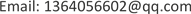FDI，金融发展，经济增长，分位数回归，通径分析, FDI Financial Development Economic Growth Quantile Regression Path Analysis
FDI与金融发展对经济增长的影响研究<sup> </sup>1. 引言

2. 文献综述

2.1. 国外研究综述

2.2. 国内研究综述

3. 研究方法3.1. 分位数回归

Roger Koenker和Gilbert Bassett在1978年提出了分位数回归的方法，与传统的回归分析不同的是，分位数回归是研究自变量与因变量之间的条件分位数之间的关系，从而得到由自变量估计因变量的条件分位数的回归模型。分位数回归不仅能得到因变量的中间趋势，还可以进一步推出因变量的条件分布概率。

F ( x ) = P ( X ≤ x ) (1)

Q τ ( X ) = arg inf { x ∈ R ; F ( x ) ≥ τ } ( 0 < τ < 1 ) (2)

F X − 1 ( τ ) = inf { y ∈ R ; F ( y ) ≥ τ } (3)

Q τ ( X ) = F X − 1 ( τ ) (4)

min β ∈ R p [ ∑ i ∈ { i : x i ≥ ξ } τ | x i − ξ | + ∑ i ∈ { i : x i < ξ } ( 1 − τ ) | x i − ξ | ] (5)

min ξ ∈ R ∑ i = 1 ρ τ ( x i − ξ ) (6)

3.2. 通径分析

1) 首先要计算出各个变量间的相关系数，

2) 根据一个因变量和多个自变量，建立以下多元回归方程：

{ P 1 y + r 12 P 2 y + ⋯ + r 1 n P n y = r 1 y r 21 P 1 y + P 2 y + ⋯ + r 2 n P n y = r 2 y ⋮ r n 1 P 1 y + r n 2 P 2 y + ⋯ + P n y = r n y (7)

3) 根据方程 r i y = P y i + ∑ P j r i j ，计算各个自变量对因变量的直接通径系数和间接通径系数，最后得出

4. FDI与金融业对经济增长的实证分析4.1. 指标选取

Indicator names and symbol

4.2. 分位数回归

Quantile regression coefficien

C1.12E−15−0.42126−0.18457−0.014000.185740.46679
x11.60101.679411.276681.702821.774062.45133
x2−1.1280−0.74186−0.95226−1.32950−1.18459−1.88169
x3−1.9300−1.91001−2.02321−1.56350−2.01341−2.39923
x40.95520.602741.158160.595130.873301.22359

5. 经济增长影响因素的通径分析5.1. 最小二乘法回归

Regression coefficient tabl

C1.12E−150.10760.00001.0000
x11.60100.46663.43200.0056
x2−1.12800.3555−3.17300.0089
x3−1.93000.3541−5.45100.0002
x40.95520.35382.70000.0207

y = 1.12 × 10 − 15 + 1.601 x 1 − 1.128 x 2 − 1.93 x 3 + 0.9552 x 4 (8)

5.2. 通径分析

Self-correlation coefficient tabl
yx1x2x3x4
y1−0.3747−0.5330−0.6003−0.3095
x1−0.374710.94020.94250.9461
x2−0.53300.940210.92510.9161
x3−0.60030.94250.925110.9048
x4−0.30950.94610.91610.90481

Path factor tabl

x1−0.37471.6010−1.9758-−1.0605−1.81900.9037
x2−0.5330−1.12800.59501.5053-−1.78540.8751
x3−0.6003−1.93001.32971.5089−1.0435-0.8643
x4−.30950.9552−1.26501.5147−1.0334−1.7463-

6. 结论与建议6.1. 结论

1) 分位数回归结果

2) 通径分析结果

FDI为我国的经济增长提供了有利的条件，且FDI对经济增长的间接影响效应大于直接影响效应。FDI对经济增长的间接影响效应中，金融发展(社会融资规模)和投资水平(固定资产投资额)两者的作用较大，而通过进出口额的影响效应较小。

6.2. 建议